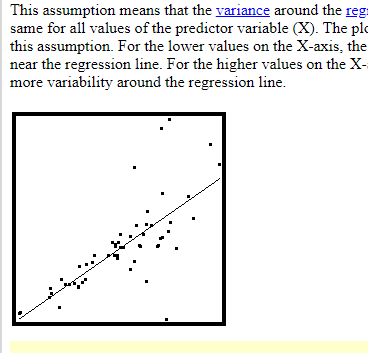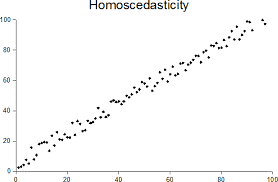# Define homoscedasticity

745    Asked by ranjan_6399 in Data Science , Asked on Jan 15, 2020

Homoscedasticity is an assumption which means that the variance around the regression line is the same for all the values of a predictor variable.In the above diagram the points are all near the regression line for the lower values on X-axis but the points are far from the line for higher values on X-axis which is a violation of this assumption.In this diagram, the variance is same throughout all the values which shows that it follows homoscedasticity.